### Integration using a System of Equations

Good morning fellow mathematicians! Today we are going to discuss an integration method, which I find to be pretty helpful and applicable in many situations. After deriving the formula we are interested in, I'm going to provide you with an example, where this very method might come in handy.

Let us consider the following integral

$$\mathcal{I}=\int\limits_{a}^{b}\frac{h(x)}{f(x)+g(x)}dx\text{ ,}$$

where $f,g,h$ are real or complex valued functions which are differentiable in the interval $[a,b]$. We would like to construct ourselves another integral $\mathcal{J}$, such that $\mathcal{I}+\mathcal{J}=(b-a)$. Let us thus define some real or complex valued function $i$, such that $h(x)+i(x)=f(x)+g(x)$ and

$$\mathcal{J}=\int\limits_{a}^{b}\frac{i(x)}{f(x)+g(x)}dx\text{ .}$$

It is now quite obvious, that $\mathcal{I}+\mathcal{J}$ is going to give us $(b-a)$, since:

\begin{align*} \mathcal{I}+\mathcal{J}&=\int\limits_{a}^{b}\frac{h(x)+i(x)}{f(x)+g(x)}dx\\ &=\int\limits_{a}^{b}\frac{f(x)+g(x)}{f(x)+g(x)}dx\\ &=\int\limits_{a}^{b}dx\\ &=(b-a)\\ \end{align*}

The first identity has thus been established. But what about $\mathcal{I}-\mathcal{J}$? Let us now place a restriction on $f(x)+g(x)$. This restriction has to do with $f$ and $g$ being differentiable by assumption (and hence also their sum) and is going to connect their sum with the other two functions $h$ and $i$. We consider the two cases:

$$\dfrac{\text{d}}{\text{d}x}[f(x)+g(x)]=\pm[h(x)-i(x)]$$

Then we get for the difference of both integrals and by introducing the substitution $t=f(x)+g(x)$, which immediately implies $\text{d}t=\pm[h(x)-i(x)]\text{d}x$:

\begin{align*} \mathcal{I}-\mathcal{J}&=\int\limits_{a}^{b}\frac{h(x)-i(x)}{f(x)+g(x)}dx\\ &=\int\limits_{f(a)+g(a)}^{f(b)+g(b)}\pm\frac{dt}{t}\\ &=\pm\text{ln}\left(\frac{f(b)+g(b)}{f(a)+g(a)}\right) \end{align*}

With those calculations out of the way, we can now add both equations together, and thus solve our system of equations to get a value for $\mathcal{I}$:

$$2\mathcal{I}=(b-a)\pm\text{ln}\left(\frac{f(b)+g(b)}{f(a)+g(a)}\right)$$
$$\iff\mathcal{I}=\frac{(b-a)}{2}\pm\frac{1}{2}\text{ln}\left(\frac{f(b)+g(b)}{f(a)+g(a)}\right)$$

That was quite some work! But we have successfully arrived at a final expression for the integral in question. Now for the applications: Let $f(x)=i(x)=\text{cos}(x)$ and $g(x)=h(x)=\text{sin}(x)$ on $[0,\frac{\pi}{2}]$. I'm leaving it as an exercise to the reader to check, if all restrictions on the functions do indeed hold in this case and that we have to choose the negative branch when doing the substitution. It does follow now, that:

\begin{align*} \mathcal{I}&=\int\limits_{0}^{\frac{\pi}{2}}\frac{\text{sin}(x)}{\text{cos}(x)+\text{sin}(x)}dx\\ &=\frac{(\frac{\pi}{2}-0)}{2}-\frac{1}{2}\text{ln}\left(\frac{\text{cos}(\frac{\pi}{2})+\text{sin}(\frac{\pi}{2})}{\text{cos}(0)+\text{sin}(0)}\right)\\ &=\frac{\pi}{4} \end{align*}

As you might notice, we were able to calculate the value of this integral pretty fast this way! Feel free to try this out using just the exponential function or other ones which satisfy the given conditions.

I hope you enjoyed the first contribution to this site and up until the next post,

--have a flammable day  $\int d \tau$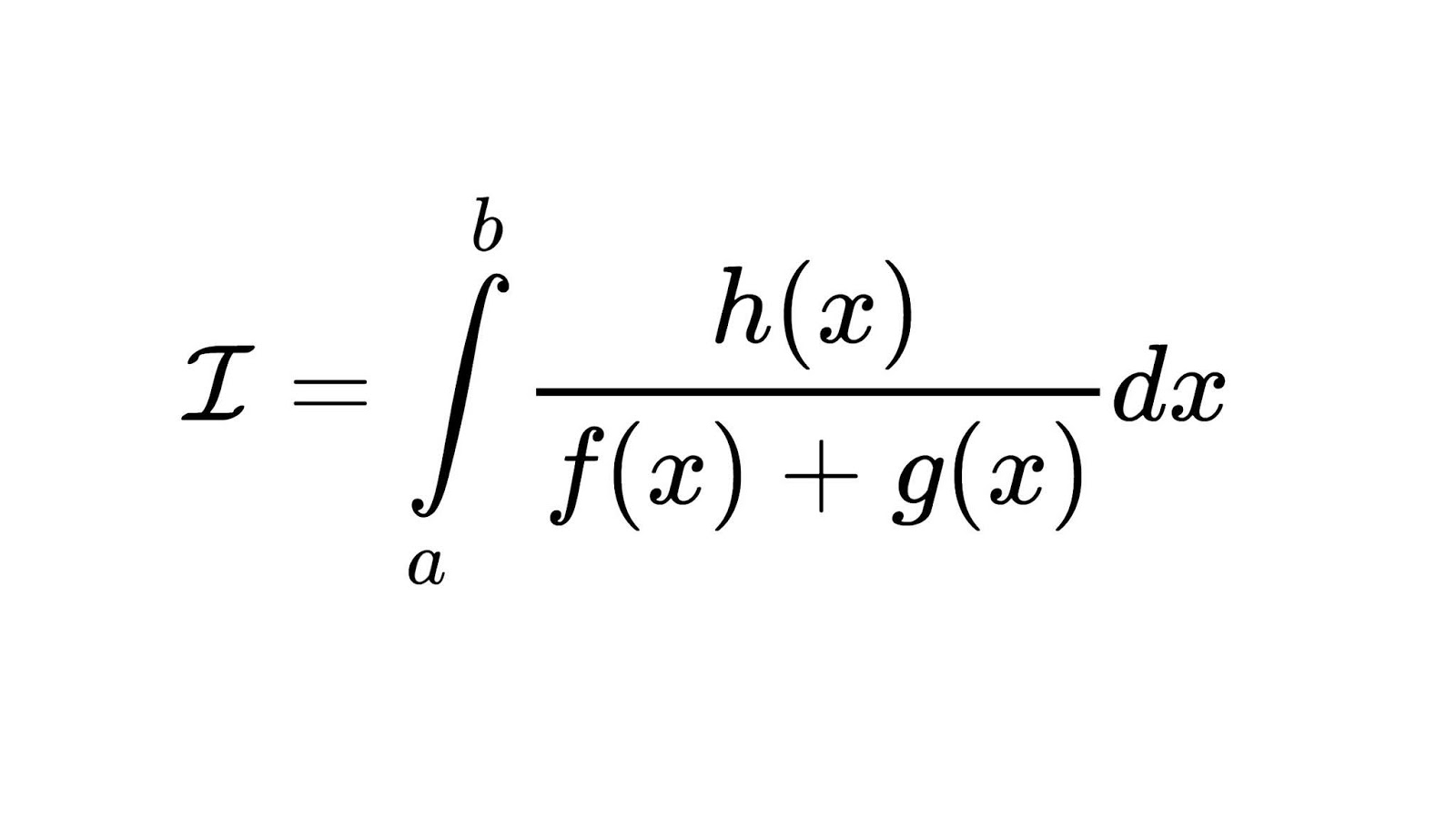1.Very nice. Love your Channel as well. Grüße aus Hannover xD

1.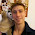2.You make math something very exciting for anyone. Amo seu canal ♡

1.3.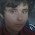1.4.Well done papa. Gonna try it with exponentials .

1.yes! It works wonders :)

5.Hi Pappa Flammy !!!! I'm a big fan and I love calculus a lot. You're a big inspiartion to me :)

1.6.Really interesting

1.7.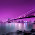Really great work!

1.thank you <3

8.Hey its Oon Han here! Nice work! Though I wonder what if we generalised to cases where the derivative of f(x) + g(x) isn't +-(h(x) - i(x))...

1.9.This comment has been removed by the author.

10.You are doing great work, problem you posted on Youtube are really unique and open new perspective to understand theories related with it>. Good job n good luck.

1.11.Thats what we call wonderful math 😍

12.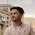jesus christ. I had my first lesson on integrals today and I was lit thankfully to your videos. But this article... I don't understand half of it, but it was interesting to read 😊

1.Enjoy the integration theory! :)

13.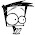A nice approach!

1.14.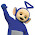Can i get a comment from Papa

1.15.Great Work! You always increment my interest in Math with your videos with all the enthusiasm you pour in. Cheers!

-Paras Khosla

1.16.This comment has been removed by the author.

17.Hi this doubt is unrelated but for the tag of integration. I wish to compute $$\int_{-\infty}^{+\infty} \dfrac{\sin x}{x(x^2+1)}\mathrm dx$$. With my choice of contour (the upper half complex plane), one the poles namely z=0 lies on its boundary. What should be my approach further? Thanks in Anticipation.

18.Outstanding!

1.19.I have penned this in to the integration chapter of my copy of K.F Riley's mathematical methods text - truly a worthy addition

20.This method would be near bulletproof if it weren't for that pesky restriction on f(x)+g(x)…

Beautiful work nonetheless!

21.